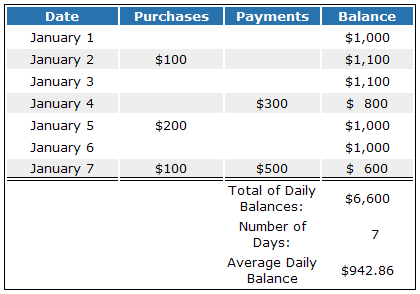## What is Average Balance?

Average balance is either the simple or the weighted average balance of a financial account during some period of time.

## How Does Average Balance Work?

A simple average balance is calculated by adding up the beginning balance and the ending balance and dividing the sum by 2. A weighted average balance takes into account the length of time a balance was at a specific level during the measurement period.

The most common use of average balance is when credit card companies use a weighted average balance to calculate finance charges. They first calculate the average daily balance and then multiply it by the appropriate interest rate.

The method for finding the average daily balance is illustrated in the chart below. In this example, let's assume the following:

Starting balance on Jan 1: \$1,000
Period length: 7 days
Purchases:
Jan 2: \$100
Jan 5: \$200
Jan 7: \$100
Payments:
Jan 4: \$300
Jan 7: \$500

Beginning with the starting balance, add the purchases and subtract the payments for each day to find that day's balance. Be sure to include all the days in the period, even those with no transaction activity.Add the daily balances and divide by the number of days in the period to find the average daily balance.

## Why Does Average Balance Matter?

Credit card companies determine your monthly finance charge by calculating the average daily balance and multiplying it by the applicable interest rate.

As you can deduce from the example above, making payments earlier on your outstanding credit balance can reduce your overall average daily balance, which, in turn, will reduce your interest payments.